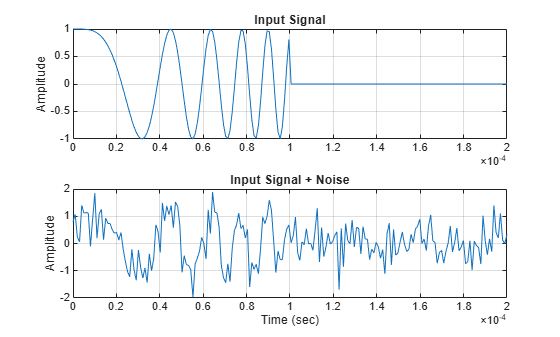## Matched Filtering

### Reasons for Using Matched Filtering

You can see from the results in Receiver Operating Characteristics that the probability of detection increases with increasing SNR. For a deterministic signal in white Gaussian noise, you can maximize the SNR at the receiver by using a filter matched to the signal. The matched filter is a time-reversed and conjugated version of the signal. The matched filter is shifted to be causal.

### Support for Matched Filtering

Use `phased.MatchedFilter` to implement a matched filter.

When you use `phased.MatchedFilter`, you can customize characteristics of the matched filter such as the matched filter coefficients and window for spectrum weighting. If you apply spectrum weighting, you can specify the coverage region and coefficient sample rate; Taylor, Chebyshev, and Kaiser windows have additional properties you can specify.

### Matched Filtering of Linear FM Waveform

This example compares the results of matched filtering with and without spectrum weighting. Spectrum weighting is often used with linear FM waveforms to reduce the sidelobes in the time domain.

Note: This example runs only in R2016b or later. If you are using an earlier release, replace each call to the function with the equivalent `step` syntax. For example, replace `myObject(x)` with `step(myObject,x)`.

Create a linear FM waveform with a duration of 0.1 milliseconds, a sweep bandwidth of 100 kHz, and a pulse repetition frequency of 5 kHz. Add noise to the linear FM pulse and filter the noisy signal using a matched filter. This example applies a matched filter with and without spectrum weighting.

Specify the waveform.

```waveform = phased.LinearFMWaveform('PulseWidth',1e-4,'PRF',5e3,... 'SampleRate',1e6,'OutputFormat','Pulses','NumPulses',1,... 'SweepBandwidth',1e5); wav = getMatchedFilter(waveform);```

Create a matched filter with no spectrum weighting, and a matched filter that uses a Taylor window for spectrum weighting.

```filter = phased.MatchedFilter('Coefficients',wav); taylorfilter = phased.MatchedFilter('Coefficients',wav,... 'SpectrumWindow','Taylor');```

Create the signal and add noise.

```sig = waveform(); rng(17) x = sig + 0.5*(randn(length(sig),1) + 1j*randn(length(sig),1));```

Filter the noisy signal separately with each of the filters.

```y = filter(x); y_taylor = taylorfilter(x);```

Plot the real parts of the waveform and noisy signal.

```t = linspace(0,numel(sig)/waveform.SampleRate,... waveform.SampleRate/waveform.PRF); subplot(2,1,1) plot(t,real(sig)) title('Input Signal') xlim([0 max(t)]) grid on ylabel('Amplitude') subplot(2,1,2) plot(t,real(x)) title('Input Signal + Noise') xlim([0 max(t)]) grid on xlabel('Time (sec)') ylabel('Amplitude')```Plot the magnitudes of the two matched filter outputs.

```plot(t,abs(y),'b--') title('Matched Filter Output') xlim([0 max(t)]) grid on hold on plot(t,abs(y_taylor),'r-') ylabel('Magnitude') xlabel('Seconds') legend('No Spectrum Weighting','Taylor Window') hold off```### Matched Filtering to Improve SNR for Target Detection

This example shows how to improve the SNR by performing matched filtering.

Note: This example runs only in R2016b or later. If you are using an earlier release, replace each call to the function with the equivalent `step` syntax. For example, replace `myObject(x)` with `step(myObject,x)`.

Place an isotropic antenna element at the global origin (0;0;0). Then, place a target with a nonfluctuating RCS of 1 square meter approximately 7 km from the transmitter at (5000;5000;10). Set the operating (carrier) frequency to 10 GHz. To simulate a monostatic radar, set the `InUseOutputPort` property on the transmitter to `true`. Calculate the range and angle from the transmitter to the target.

```antenna = phased.IsotropicAntennaElement('FrequencyRange',[5e9 15e9]); transmitter = phased.Transmitter('Gain',20,'InUseOutputPort',true); fc = 10e9; target = phased.RadarTarget('Model','Nonfluctuating',... 'MeanRCS',1,'OperatingFrequency',fc); txloc = [0;0;0]; tgtloc = [5000;5000;10]; transmitterplatform = phased.Platform('InitialPosition',txloc); targetplatform = phased.Platform('InitialPosition',tgtloc); [tgtrng,tgtang] = rangeangle(targetplatform.InitialPosition,... transmitterplatform.InitialPosition);```

Create a rectangular pulse waveform 25 μs in duration with a PRF of 10 kHz. Use a single pulse for this example. Determine the maximum unambiguous range for the given PRF. Use the `radareqpow` function to determine the peak power required to detect a target. This target has an RCS of 1 square meter at the maximum unambiguous range for the transmitter operating frequency and gain. The SNR is based on a desired false-alarm rate of 1e-6 for a noncoherent detector.

```waveform = phased.RectangularWaveform('PulseWidth',25e-6,... 'OutputFormat','Pulses','PRF',10e3,'NumPulses',1); c = physconst('LightSpeed'); maxrange = c/(2*waveform.PRF); SNR = npwgnthresh(1e-6,1,'noncoherent'); Pt = radareqpow(c/fc,maxrange,SNR,... waveform.PulseWidth,'RCS',target.MeanRCS,'Gain',transmitter.Gain);```

Set the peak transmit power to the output value from `radareqpow`.

`transmitter.PeakPower = Pt;`

Create radiator and collector objects that operate at 10 GHz. Create a free space path for the propagation of the pulse to and from the target. Then, create a receiver and a matched filter for the rectangular waveform.

```radiator = phased.Radiator('PropagationSpeed',c,... 'OperatingFrequency',fc,'Sensor',antenna); channel = phased.FreeSpace('PropagationSpeed',c,... 'OperatingFrequency',fc,'TwoWayPropagation',false); collector = phased.Collector('PropagationSpeed',c,... 'OperatingFrequency',fc,'Sensor',antenna); receiver = phased.ReceiverPreamp('NoiseFigure',0,... 'EnableInputPort',true,'SeedSource','Property','Seed',2e3); filter = phased.MatchedFilter(... 'Coefficients',getMatchedFilter(waveform),... 'GainOutputPort',true);```

After you create all the objects that define your model, you can propagate the pulse to and from the target. Collect the echo at the receiver, and implement the matched filter to improve the SNR.

Generate waveform.

`wf = waveform();`

Transmit waveform.

`[wf,txstatus] = transmitter(wf);`

`wf = radiator(wf,tgtang);`

Propagate pulse toward the target.

`wf = channel(wf,txloc,tgtloc,[0;0;0],[0;0;0]);`

Reflect it off the target.

`wf = target(wf);`

Propagate the pulse back to transmitter.

`wf = channel(wf,tgtloc,txloc,[0;0;0],[0;0;0]);`

Collect the echo.

`wf = collector(wf,tgtang);`

```rx_puls = receiver(wf,~txstatus); [mf_puls,mfgain] = filter(rx_puls);```

Get group delay of matched filter.

`Gd = length(filter.Coefficients)-1;`

The group delay is constant.

Shift the matched filter output.

```mf_puls=[mf_puls(Gd+1:end); mf_puls(1:Gd)]; subplot(2,1,1) t = unigrid(0,1e-6,1e-4,'[)'); rangegates = c.*t; rangegates = rangegates/2; plot(rangegates,abs(rx_puls)) title('Received Pulse') ylabel('Amplitude') hold on plot([tgtrng, tgtrng], [0 max(abs(rx_puls))],'r') subplot(2,1,2) plot(rangegates,abs(mf_puls)) title('With Matched Filtering') xlabel('Meters') ylabel('Amplitude') hold on plot([tgtrng, tgtrng], [0 max(abs(mf_puls))],'r') hold off```